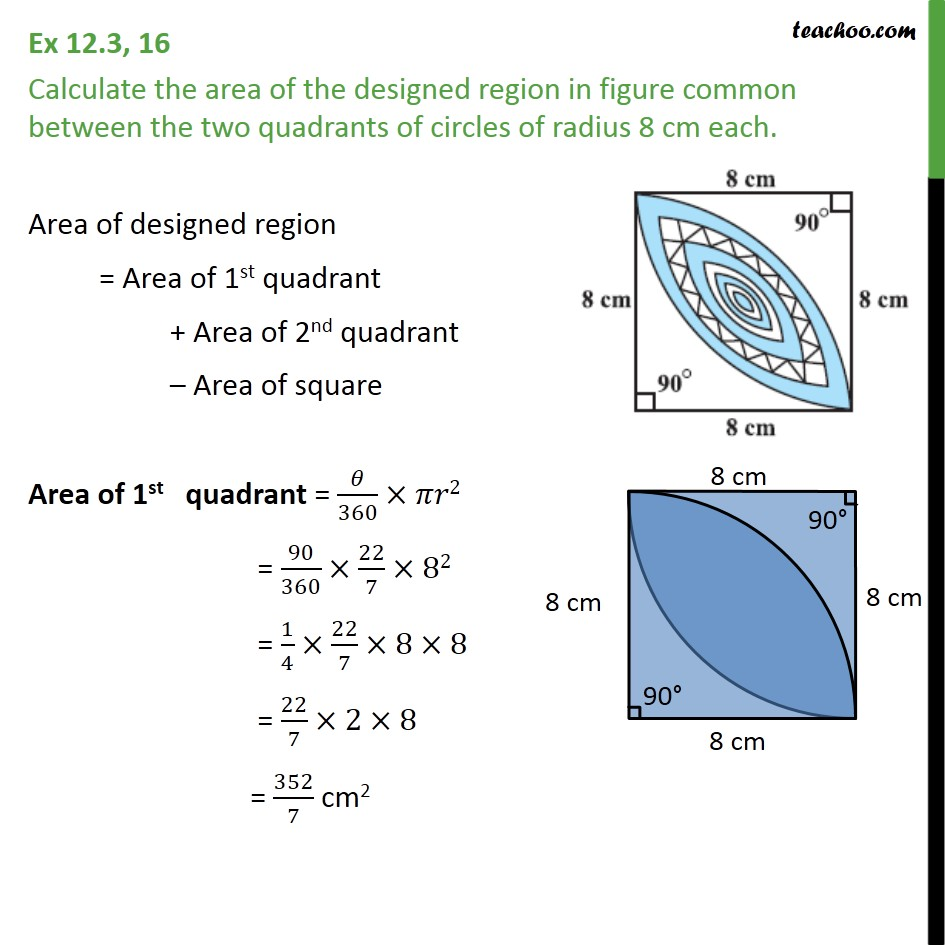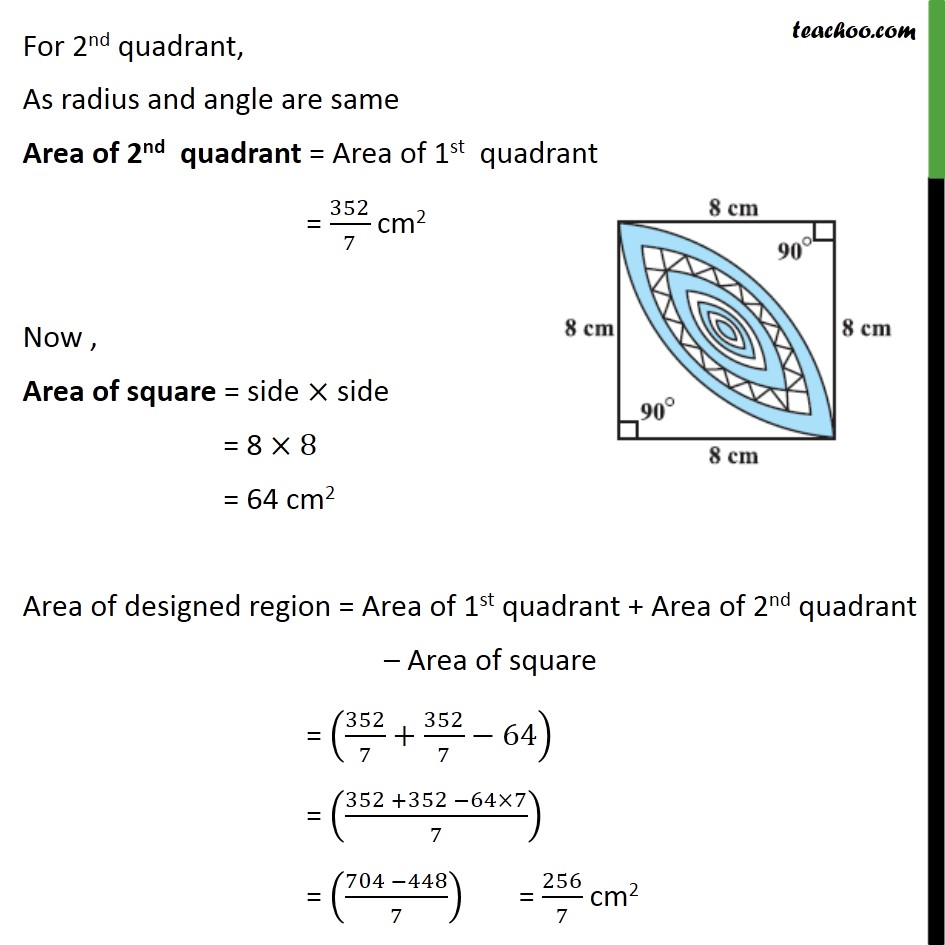1. Chapter 12 Class 10 Areas related to Circles (Term 1)
2. Serial order wise
3. Ex 12.3

Transcript

Ex 12.3, 16 Calculate the area of the designed region in figure common between the two quadrants of circles of radius 8 cm each. Area of designed region = Area of 1st quadrant + Area of 2nd quadrant Area of square Area of 1st quadrant = /360 2 = 90/360 22/7 82 = 1/4 22/7 8 8 = 22/7 2 8 = 352/7 cm2 For 2nd quadrant, As radius and angle are same Area of 2nd quadrant = Area of 1st quadrant = 352/7 cm2 Now , Area of square = side side = 8 8 = 64 cm2 Area of designed region = Area of 1st quadrant + Area of 2nd quadrant Area of square = (352/7+352/7 64) = ((352 +352 64 7)/7) = ((704 448)/7) = 256/7 cm2